• 数据分析-数据平滑处理 数据平滑处理 在做数据分析时, 由于数据的噪声太多, 需要对数据进行数据平滑处理. 通常包含有降噪/拟合等操作. 降噪的功能在于去除额外的影响因素. 拟合的目的在于数学模型化,可以通过更多的...
数据分析-数据平滑处理
数据平滑处理
在做数据分析时, 由于数据的噪声太多, 需要对数据进行数据平滑处理. 通常包含有降噪/拟合等操作. 降噪的功能在于去除额外的影响因素. 拟合的目的在于数学模型化,可以通过更多的数学方法识别曲线特征.
降噪手段: 卷积运算
# 通常卷积核的选取API
con_core = np.hanning(8)
con_core /= con_core.sum()
# 卷积API
np.convolve(samples, con_core, 'valid')

数学模型化: 多项式拟合
# 通过原函数曲线(x,y)/最高次数 得到 拟合多项式系数
P = np.polyfit(x, y, n)
# 通过多项式系数与x的值,求对应的多项式函数值.
y = np.polyval(P, x)
# 通过原函数求导函数
Q = np.polyder(P)
# 求多项式的根
X = np.roots(Q)
# 求两个多项式函数的差函数. 可以通过该方法求取两个多项式
# 函数的交点
Q = np.polysub(P1, P2)

符号数组
sign函数可以把样本数组变成对应的符号数组, 所有正数变为1, 负数变为-1, 0还是0.
ary = np.sign(源数组)

绘制 净额成交量(OBV)
若相比上一天的收盘价上涨,则为正成交量; 若比上一天的收盘价下跌,则为负成交量.
成交量可以反映市场对某支股票的人气, 成交量是一支股票上涨的能量. 一般情况下股票上涨往往需要较大的成交量,而下跌时则不然.
案例: 绘制OBV柱状图
import numpy as np
import matplotlib.pyplot as mp
import datetime as dt
import matplotlib.dates as md

def dmy2ymd(dmy):
dmy = str(dmy, encoding='utf-8')
date = dt.datetime.strptime(
dmy, '%d-%m-%Y').date()
s = date.strftime("%Y-%m-%d")
return s

# 加载文件
'../data/bhp.csv', delimiter=',',
usecols=(1, 6, 7), unpack=True,
dtype='M8[D], f8, f8',
converters={1: dmy2ymd})

# 获取相比上一天股价是否上涨
diff_closing_prices = np.diff(closing_prices)
# 获取相对应的符号数组
sign_closing_prices = np.sign(diff_closing_prices)
# 绘制每天的成交量
obvs = volumes[1:] * sign_closing_prices

# 绘制净额成交量柱状图
mp.figure('OBV', facecolor='lightgray')
mp.title('OBV', fontsize=18)
mp.xlabel('Dates', fontsize=14)
mp.ylabel('Volumes', fontsize=14)
# 整理x轴刻度定位器
ax = mp.gca()
ax.xaxis.set_major_locator(
md.WeekdayLocator(byweekday=md.MO))
ax.xaxis.set_major_formatter(
md.DateFormatter('%d %b %Y'))
ax.xaxis.set_minor_locator(md.DayLocator())

mp.tick_params(labelsize=10)
mp.grid(linestyle=':', axis='y')
dates = dates[1:].astype(md.datetime.datetime)

mp.bar(dates, obvs, 1.0, color='dodgerblue',
edgecolor='white', label='OBV')

mp.gcf().autofmt_xdate()
mp.legend()
mp.show()

数组预处理函数
# array 源数组
# 条件序列:  [a<60, a==60, a>60]
# 取值序列:  [-1,   0,     1   ]
np.piecewise(array, 条件序列, 取值序列)

测试:
a = np.array([23, 94, 65, 23, 84, 56, 23])
d = np.piecewise(
a,
[(20 < a) & (a < 60), a == 60, a > 60],
[-1, 0, 1])
print(d)

矢量化
矢量化指的是用数组代理标量来操作数组里的每个元素.
numpy提供了vectorize函数, 可以把处理标量的函数矢量化. 经过vectorize函数矢量化处理过后将会返回一个矢量函数, 该函数可以直接处理ndarray数组.
案例:
import numpy as np
import math as m

def foo(x, y):
return m.sqrt(x**2 + y**2)

x, y = 3, 4
print(foo(x, y))
x = np.array([3, 4, 5, 6])
y = np.array([4, 5, 6, 7])
# z = foo(x, y)  错误
# 把foo函数矢量化处理
foo_v = np.vectorize(foo)
print(foo_v(x, y))

numpy提供了frompyfunc函数, 也可以完成vectorize相同的功能
# 使用frompyfunc方法矢量化函数
# foo需要2个参数, 最终将会有1个返回值
foo_f = np.frompyfunc(foo, 2, 1)
print(foo_f(x, y))

矩阵
numpy.matrix类型用来描述矩阵. 该类继承自numpy.ndarray. 任何多维数组的操作,对矩阵同样有效. 但是作为子类矩阵又结合了自身的特点,做了必要的扩充,比如乘法计算/矩阵求逆等等.
矩阵的创建
# ary: 任何可以被解释为矩阵的二维容器
# copy: 如果copy的值为True(缺省), 所得到的矩阵对象与参
# 数中源容器各自拥有独立的数据拷贝.否则,共享同一份数据
numpy.matrix(ary, copy=True)

# ary: 任何可以被解释为矩阵的二维容器
# 默认copy=False
numpy.mat(ary)

# str: 字符串矩阵拼块规则
#      '1 2 3;4 5 6;7 8 9'
np.mat(str)

矩阵的乘法运算
'''
a * b
矩阵的乘法:a矩阵的每一行分别乘以b矩阵的每一列
结果矩阵的行数与a的行数一致, 结果矩阵的列数与b的列数一致
'''
e = np.mat('1 2 6; 3 5 7; 4 8 9')
print(e * e)

矩阵的逆矩阵
若两个矩阵A/B满足: AB = BA = E (单位矩阵),则 A/B互为逆矩阵.
单位矩阵: 矩阵的主对角线值为1, 其他位置值为0的矩阵.
e = np.mat(..)
print(e.I)  #逆矩阵

案例 : 春游, 去程做大巴, 小孩票价3元, 家长票价3.2元, 一共花了118.4; 回程做火车, 小孩3.5, 家长3.6, 花了135.2; 求小孩与家长的人数.
\left[ \begin{array}{ccc}
x & y
\end{array} \right]
\times
\left[ \begin{array}{ccc}
3  & 3.5 \
3.2 & 3.6 \
\end{array} \right]
\left[ \begin{array}{ccc}
118.4 & 135.2
\end{array} \right]
斐波那契数列
1 1 2 3 5 8 13 ...

1 1  1 1  1 1  1 1
X  1 0  1 0  1 0  1 0
---------------------------------------------
1 1  2 1  3 2  5 3
1 0  1 1  2 1  3 2  ...

numpy通用函数
加法通用函数
np.add(a, b)			# 两数组相加

案例:
a = np.arange(1, 7)
print(a)

除法通用函数
# 真除
a / b
np.true_divide(a, b)
np.divide(a, b)
# 地板除
np.floor_divide(a, b)
np.ceil(a / b)	# 天花板除
np.trunc(a / b) # 截断除

import numpy as np

a = np.array([20, 20, -20, -20])
b = np.array([3, -3, 6, -6])
print(a)
print(b)
# 开始测试
print(np.true_divide(a, b))
print(np.divide(a, b))

print(np.floor_divide(a, b))
print(np.ceil(a / b))
print(np.trunc(a / b))

三角函数通用函数
np.sin()  np.cos()

傅里叶定理
傅里叶说过, 任何周期函数都可以由多个不同振幅/频率/相位的正弦函数叠加而成.
合成方波
一个方波由如下参数的正弦波叠加而成:
y = 4\pi \times sin(x) \
y = \frac{4}{3}\pi \times sin(3x) \
y = \frac{4}{5}\pi \times sin(5x) \
y = \frac{4}{7}\pi \times sin(7x) \
… \
y = \frac{4}{2n-1}\pi \times sin((2n-1)x) \
案例:
import numpy as np
import matplotlib.pyplot as mp

x = np.linspace(-2 * np.pi, 2 * np.pi, 1000)
# 根据公式搞出来3条曲线
y1 = 4 * np.pi * np.sin(x)
y2 = 4 / 3 * np.pi * np.sin(3 * x)
y3 = 4 / 5 * np.pi * np.sin(5 * x)

# 使用循环控制叠加波的数量
n = 1000
y = np.zeros(1000)
for i in range(1, n + 1):
y += 4 / (2 * i - 1) * np.pi * \
np.sin((2 * i - 1) * x)

mp.figure('SIN', facecolor='lightgray')
mp.title('SIN', fontsize=18)
mp.xlabel('X', fontsize=14)
mp.ylabel('Y', fontsize=14)
mp.tick_params(labelsize=10)

mp.plot(x, y1, label='y1')
mp.plot(x, y2, label='y2')
mp.plot(x, y3, label='y3')
mp.plot(x, y, label='y')

mp.legend()
mp.show()

位运算通用函数
位异或
c = a ^ b
c = np.bitwise_xor(a, b)

按位异或可以很方便的判断两个数据是否同号
a = np.array([4, -6, 7, -3, -4, 2])
b = np.array([-2, -8, 2, -5, 3, -4])

print(a)
print(b)
print(a ^ b)
print(np.bitwise_xor(a, b))
# where找到符合条件的元素下标 (异号)
print(np.where((a ^ b) < 0))

位与
e = a & b
e = np.bitwise_and(a, b)

利用位与运算计算某个数字是否是2的幂
'''
1  2^0  00001    0  00000
2  2^1  00010    1  00001
4  2^2  00100    3  00011
8  2^3  01000    7  00111
'''
print('-' * 40)
d = np.arange(1, 20)
print(d)
e = np.bitwise_and(d, d - 1)
print(e)

位或 / 位反 / 移位
# 位或操作
np.bitwise_or(a, b)
# 位反操作 (1变0, 0变1)
np.bitwise_not(a)
# 移位操作
np.left_shift(array, 1)		#每个元素左移1位 (乘2)
np.right_shift(array, 1)	#每个元素右移1位 (除2)

numpy提供的线性代数模块(linalg)
逆矩阵和广义逆矩阵
如果一个方阵A与方阵B的乘积是单位矩阵,则AB互为逆矩阵.
np.linalg.inv(A)  # 返回A方阵的逆矩阵

如果A不是方阵, A的逆矩阵则称为广义逆矩阵.
np.linalg.pinv(A) # 返回矩阵A的广义逆矩阵

d = A.I # 既可以返回逆矩阵也可以返回广义逆矩阵

线性方程求解与线性拟合
# 求解线性方程组
c = np.linalg.solve(a, b)
# 线性拟合(求出误差最小的结果矩阵)
c = np.linalg.lstsq(a, b)

\begin{cases}
x -2y+z=0\
2y-8z=8\
-4x+5y+9z=-9
\end{cases}


展开全文• ## 10.数据平滑处理

万次阅读 2017-09-19 15:32:22
我们在上一篇文章中可以看到,我们的数据是不平滑的,这对拟合来说就有影响,而且有的噪声数据会影响拟合的函数的准确性.所以在对数据拟合前,应该进行平滑 移动平均线和窗函数
我们在上一篇文章中可以看到,我们的数据是不平滑的,这对拟合来说就有影响,而且有的噪声数据会影响拟合的函数的准确性.所以在对数据拟合前,应该进行平滑处理

简单移动平均线

简单移动平均线是计算与等权重的指示函数的卷积,也可以不等权重.
1.用ones函数创建一个元素均为1的数组,然后对整个数组除以N,得到等权重.
2.使用权值,调用convolve函数.
3.从convolve函数分安徽的数组中取出中间的长度为N的部分(即两者作卷积运算时完全重叠的区域.)
4.使用matplotlib绘图

import numpy as np
import matplotlib.pyplot as plt
import sys

N=int(sys.argv)
weights=np.ones(N)/N
print("WEIGHTS",weights)

skiprows=(2),usecols=(2,),unpack=True)
sam=np.convolve(weights,c)[N-1:-N+1]
t=np.arange(N-1,len(c))

plt.plot(t,c[N-1:],lw=1.0)
plt.plot(t,sam,lw=2.0)
plt.show()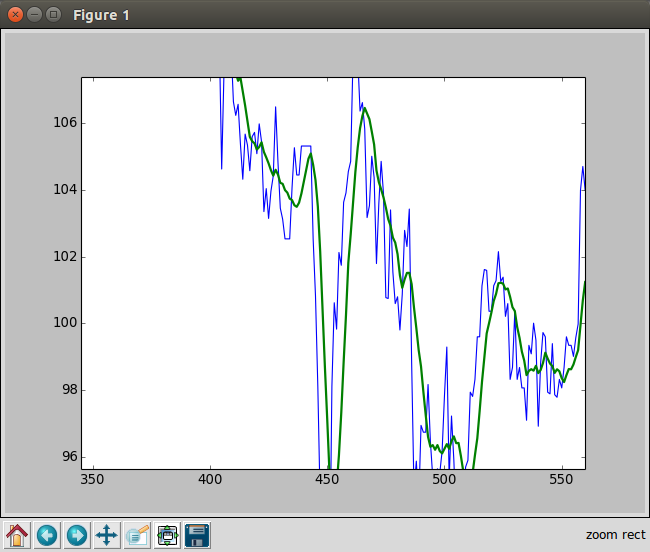窗函数:hanning汉宁窗

汉宁窗是一个加权余弦窗函数.numpy.hanning(M) Return the Hanning window.

Parameters:    M : int
Number of points in the output window. If zero or
Returns:  out : ndarray, shape(M,)
The window, with the maximum value normalized to one (the value one
appears only if M is odd).

1.调用hanning函数计算权重,生成一个长度为N的窗口,输入参数N

N=int(sys.argv)
weights=np.hanning(N)
print(weights)

2.使用convolve,进行卷积运算.然后绘图.

import numpy as np
import matplotlib.pyplot as plt
import sys

N=int(sys.argv)
weights=np.hanning(N)
print("WEIGHTS",weights)

skiprows=(2),usecols=(2,),unpack=True)
sam=np.convolve(weights/weights.sum(),c)[N-1:-N+1]
t=np.arange(N-1,len(c))

plt.plot(t,c[N-1:],lw=1.0)
plt.plot(t,sam,lw=2.0)
plt.show()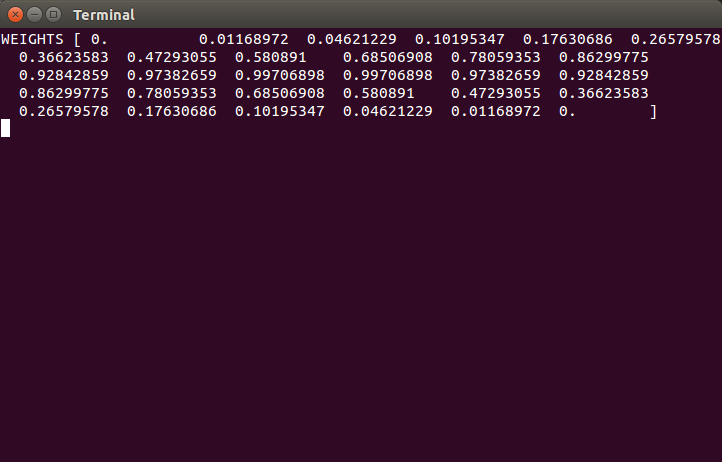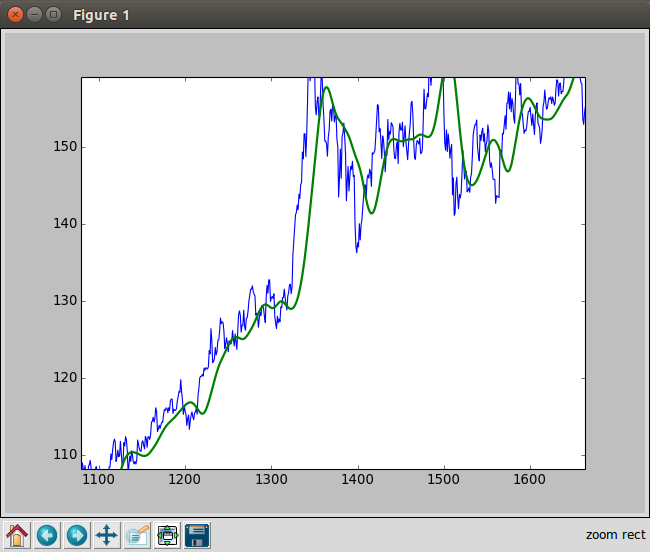展开全文移动平均线
在自然语言处理中，经常要计算单词序列（句子）出现的概率估计。但是，算法训练的时候，预料库中不可能包含所有可能出现的序列，因此为了防止对训练样本中为出现的新序列概率估计值为零，人们发明了不少可以改善估计新序列出现的概率算法，即数据的平滑。最常见的数据平滑算法包括如下几种：

Backoff回退法

Interpolation插值法

Absolute discounting

Kneser-Ney smoothing

Modified Kneser-ney smoothing

这几个方法实际上可以简单的理解为三种不同的方法：第一种类型为政府给大家每人一笔或者几笔钱（如1和2），第二种为找父母要（如3和4），最后一种就是劫富济贫（如5-7）。下面依次简单介绍上面的方法，具体详细的介绍，大家可以参阅相应的论文和书籍。

数据预处理

在介绍上面几种平滑的方法之前，这里先给出一个简单的的数据预处理的方法，特别是对于OOV（需要训练的词不在词袋里面）的情况特别有效，而且如果训练的时候，如果有几十万的词汇，一般不会对这几十万的词汇进行全部训练，而是需要预先做下面的处理后再进行数据的平滑和训练。

假设训练数据集中出现了|N|个不同的词汇，那么可以根据词频对这些词汇进行排序，可以选择词频最高的M个词汇作为我们的词汇集合，这样在训练和测试数据集中，将不属于V的词汇都替换成特殊的词汇UNK，这样可以大大减少计算量，也可以提高计算的精度。

于是对于n-gram的模型而言，假设V是所有可能的不同的N-gram的类型个数，那么根据贝叶斯公式有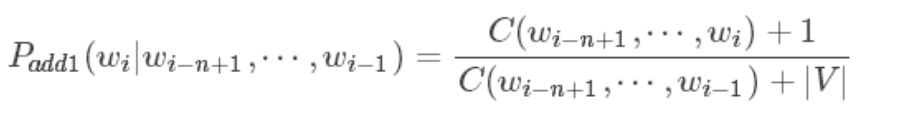当然这里的n-gram的可以相应的改成uingram和bigram表达式，并不影响。其中C（x）为x在训练中出现的次数，wi为给定的训练数据中第i个单词。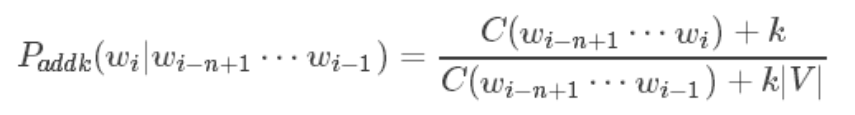Backoff回退法

回退模型，思路实际上是：如果你自己有钱，那么就自己出钱，如果你自己没有钱，那么就你爸爸出，如果你爸爸没有钱，就你爷爷出，举一个例子，当使用Trigram的时候，如果Count（trigram）满足条件就使用，否则使用Bigram，再不然就使用Unigram.

它也被称为：Katz smoothing，具体的可以去查看相应的书籍。

它的表达式为：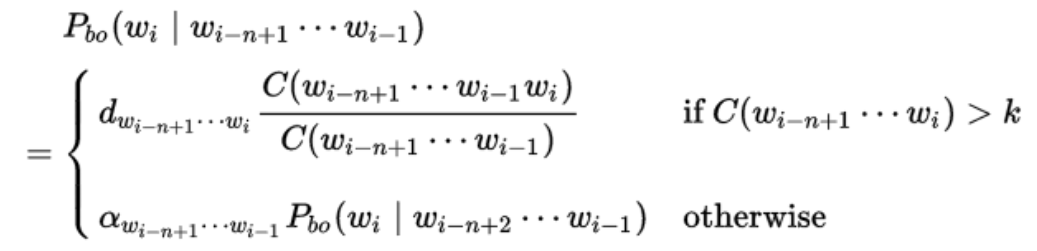其中d，a和k分别为参数。k一般选择为0，但是也可以选其它的值。

Interpolation插值法

插值法和回退法的思想非常相似，设想对于一个trigram的模型，我们要统计语料库中“”“I like chinese food”出现的次数，结果发现它没有出现，则计数为0，在回退策略中们将会试着用低阶的gram来进行替代，也就是用“like chinese food”出现的次数来替代。在使用插值的时候，我们把不同阶层的n-gram的模型线性叠加组合起来之后再使用，简单的如trigram的模型，按照如下的方式进行叠加：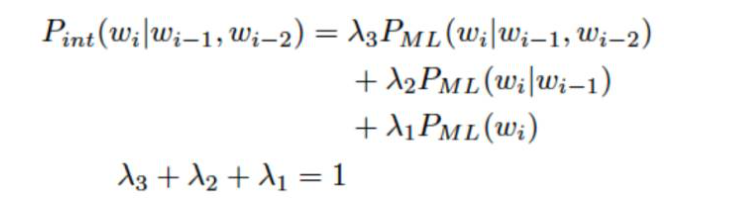参数可以凭借经验进行设定，也可以通过特定的算法来进行确定，比如EM算法。对于数据一般可以分为: traning set, development set, testing set. 那么P的概率使用training set进行训练得出，lamda参数使用development set得到。

Absolute discounting

插值法使用的参数实际上没有特定的选择，如果将lamda参数根据上下文进行选择的话就会演变成Absolute discounting。对于这个算法的基本想法是，有钱的，每个人交固定的税D，建立一个基金，没有钱的根据自己的爸爸有多少钱分这个基金。比如对于bigram的模型来说，有如下公式。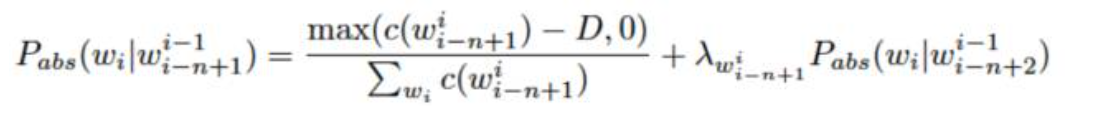D为参数，可以通过测试优化设定。

Kneser-Ney smoothing

这种算法是目前一种标准的而且是非常先进的平滑算法，它其实相当于前面讲过的几种算法的综合。它的思想实际上是：有钱的人，每个人交一个固定的税D，大家一起建立一个基金，没有钱的呢，根据自己的的爸爸的“交际的广泛”的程度来分了这个基金。这里交际的广泛实际上是指它爸爸会有多少种不同的类型，类型越多，这说明越好。其定义式为：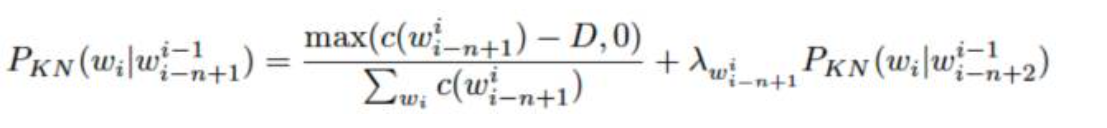其中max（c(X)-D,0）的意思是要保证最后的计数在减去一个D后不会变成一个负数，D一般大于0小于1。这个公式递归的进行，直到对于Unigram的时候停止。而lamda是一个正则化的常量，用于分配之前的概率值（也就是从高频词汇中减去的准备分配给哪些未出现的低频词的概率值（分基金池里面的基金））。其表达是为：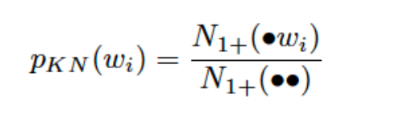PKN是在wi固定的情况下，unigram和bigram数目的比值，这里需要注意的是PKN是一个分布，它是一个非负的值，求和的话为1。

Modified Kneser-ney smoothing

这一种方法是上一种方法的改进版，而且也是现在最优的方法。上一个方法，每一个有钱的人都交一个固定的锐，这个必然会出现问题，就像国家收税一样，你有100万和你有1个亿交税的量肯定不一样这样才是比较合理的，因此将上一种方法改进就是：有钱的每个人根据自己的收入不同交不同的税D，建立一个基金，没有钱的，根据自己的爸爸交际的广泛程度来分配基金。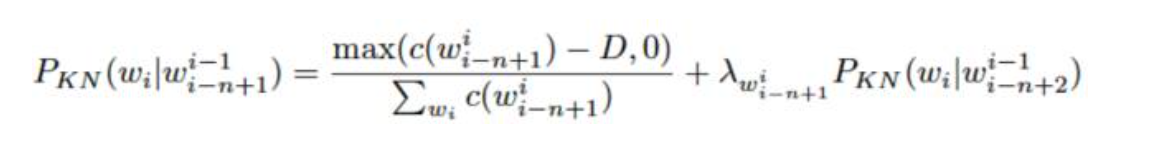这里D根据c来设定不同的值，比如c为unigram，则使用D1，c位bigram，则使用D2，如果是大于等于3阶的使用D3.

转自：微信公众号：自然语言处理技术

参考书籍：

 Speech and language processing, Daniel Jurafsky, et la.

 语音识别实践，俞栋等人。
展开全文• ## MATLAB中数据平滑处理

万次阅读 多人点赞 2017-05-05 14:31:40
smoothts函数 调用格式： output = smoothts(input) output = smoothts(input, ‘b’, ...output = smoothts(input, ‘g’, wsize, stdev) % 高斯窗方法 output = smoothts(input, ‘e’, n) % 指数法 【例7.


smoothts函数

调用格式：

output = smoothts(input)

output = smoothts(input, ‘b’, wsize) % 盒子法

output = smoothts(input, ‘g’, wsize, stdev) % 高斯窗方法

output = smoothts(input, ‘e’, n) % 指数法

【例7.1-2】现有上海股市日开盘价、最高价、最低价、收盘价、收益率等数据，时间跨度为2005年1月4日至2007年4月3日，共510组数据。完整数据保存在文件examp7_1_2.xls中，其中部分数据如下图所示。试调用smoothts函数对日收盘价数据进行平滑处理

绘制日收盘价曲线图：

% 从文件examp7_1_2.xls中读取数据

>> price = x(:,4)'; % 提取矩阵x的第4列数据，即收盘价数据

>> figure; % 新建一个图形窗口% 绘制日收盘价曲线图，黑色实线，线宽为2

>> plot(price,'k','LineWidth',2); % 为X轴和Y轴加标签

>> xlabel('观测序号');

>> ylabel('上海股市日收盘价');盒子法：

% 用盒子法平滑数据，窗宽为30

>> output1 = smoothts(price,'b',30);

% 用盒子法平滑数据，窗宽为100

>> output2 = smoothts(price,'b',100);

>> figure; % 新建一个图形窗口

>> plot(price,'.'); % 绘制日收盘价散点图

>> hold on

% 绘制平滑后曲线图，黑色实线，线宽为2

>> plot(output1,'k','LineWidth',2);

% 绘制平滑后曲线图，黑色点划线，线宽为2

>> plot(output2,'k-.','LineWidth',2);

% 为X轴和Y轴加标签

>> xlabel('观测序号'); ylabel('Box method');

% 为图形加标注框

>> legend('原始散点','平滑曲线(窗宽30)','平滑曲线(窗宽100)','location','northwest');用高斯窗方法平滑数据：

>> output3 = smoothts(price,'g',30); % 窗宽为30，标准差为默认值0.65

>> output4 = smoothts(price,'g',100,100); % 窗宽为100，标准差为100

>> figure; % 新建一个图形窗口

>> plot(price,'.'); % 绘制日收盘价散点图

>> hold on % 绘制平滑后曲线图，黑色实线，线宽为2

>> plot(output3,'k','LineWidth',2); % 绘制平滑后曲线图，黑色点划线，线宽为2

>> plot(output4,'k-.','LineWidth',2); % 为X轴和Y轴加标签>> xlabel('观测序号'); ylabel('Gaussian window method');

>> legend('原始散点','平滑曲线(窗宽30，标准差0.65)',... '平滑曲线(窗宽100，标准差100)','location','northwest');用指数法平滑数据：

>> output5 = smoothts(price,'e',30); % 用指数法平滑数据，窗宽为30

>> output6 = smoothts(price,'e',100); % 用指数法平滑数据，窗宽为100 >> figure; % 新建一个图形窗口

>> plot(price,'.'); % 绘制日收盘价散点图

>> hold on % 绘制平滑后曲线图，黑色实线，线宽为2

>> plot(output5,'k','LineWidth',2); % 绘制平滑后曲线图，黑色点划线，线宽为2

>> plot(output6,'k-.','LineWidth',2); % 为X轴和Y轴加标签>> xlabel('观测序号'); ylabel('Exponential method');

>> legend('原始散点','平滑曲线(窗宽30)','平滑曲线(窗宽100)','location','northwest');三、medfilt1函数（一维中值滤波）

调用格式：

y = medfilt1(x,n)

y = medfilt1(x,n,blksz)

y = medfilt1(x,n,blksz,dim)

【例7.1-3】产生一列正弦波信号，加入噪声信号，然后调用medfilt1函数对加入噪声的正弦波进行滤波（平滑处理）

% 产生一个从0到2*pi的向量，长度为500

>> t = linspace(0,2*pi,500)';

>> y = 100*sin(t); % 产生正弦波信号

% 产生500行1列的服从N(0,152)分布的随机数，作为噪声信号

>> noise = normrnd(0,15,500,1);

>> y = y + noise; % 将正弦波信号加入噪声信号

>> figure; % 新建一个图形窗口

>> plot(t,y); % 绘制加噪波形图

>> xlabel('t'); % 为X轴加标签

>> ylabel('y = sin(t) + 噪声'); % 为Y轴加标签中值滤波：

% 调用medfilt1对加噪正弦波信号y进行中值滤波，并绘制波形图

>> yy = medfilt1(y,30); % 指定窗宽为30，对y进行中值滤波

>> figure; % 新建一个图形窗口>> plot(t,y,'k:'); % 绘制加噪波形图

>> hold on % 绘制平滑后曲线图，黑色实线，线宽为3

>> plot(t,yy,'k','LineWidth',3);

>> xlabel('t'); % 为X轴加标签

>> ylabel('中值滤波'); % 为Y轴加标签

>> legend('加噪波形','平滑后波形');展开全文MATLAB
• 作者：cartzhang数据平滑处理由于需要处理硬件的传给数据，硬件是其他商家的。 坑爹的是发送频率太低了，20HZ左右，也是服气了。好处有么，肯定是便宜。 这个用在VR游戏上，不做评论了。 既然这样，算法
• 数据平滑：通常包含降噪、拟合等操作。降噪的功能在于去除额外的影响因素，拟合的目的在于数学模型化，可以通过更多的数学方法识别曲线的特征。 2.绘制两支股票的收益率 ---收益率 =（后一天的收盘价 - 前一天的...
• ## 几种平滑处理方法

万次阅读 2017-12-07 15:40:53
平滑，也可叫滤波，或者合在一起叫平滑滤波，平滑滤波是低频增强的空间域滤波技术。它的目的有两类：一类是模糊；另一类是消除噪音。空间域的平滑滤波一般采用简单平均法...“平滑处理”也称“模糊处理”(blurring)，
• 本文介绍了常见的信号平滑处理方法：（一阶滤波，互补滤波，卡尔曼滤波）低通滤波 互补滤波 卡尔曼滤波
• 简单的数据平滑处理方法。在一本老版本的《数学手册》中找到了几个基于最小二乘法的数据平滑算法。将其写成了C 代码，测试了一下，效果还可以。这里简单的记录一下，算是给自己做个笔记。算法的原理很简单，以五点...
• ## 信号平滑处理方法

千次阅读 2019-12-13 16:41:47
调用格式：output = smoothts(input)output = smoothts(input, ‘b’, wsize) % 盒子法output = smoothts(input, ‘g’, wsize, stdev) % 高斯窗方法output = smoothts(input, ‘e’, n) % 指数法【例7.1-2】现有...
• ## 几个简单的数据点平滑处理算法

万次阅读 多人点赞 2013-09-05 13:29:54
因此去找了些简单的数据平滑处理方法。 在一本老版本的《数学手册》中找到了几个基于最小二乘法的数据平滑算法。将其写成了C 代码，测试了一下，效果还可以。这里简单的记录一下，算是给自己做个笔记。
• 1、smooth函数平滑处理 （1）yy=smooth（y） 利用移动平均滤波器对列向量y进行平滑处理，返回与y等长的列向量yy。移动平均滤波器的默认窗宽为5，yy中元素的计算方法如下： yy（1）=y（1） yy（2）=（y（1）+y（2...
• python 数据、曲线平滑处理——方法总结Savitzky-Golay 滤波器实现曲线平滑插值法对折线进行平滑曲线处理基于Numpy.convolve实现滑动平均滤波数据平滑处理——log()和exp()函数 问题描述： 在寻找曲线的波峰、波谷时...
• smooth函数、imfilter滤波、直接用conv2，最简单的低通比如1/9*ones(3) ...%-----------------------------------------------------------------------...% 调用smooth函数进行加噪数据平滑处理 %----------
• 如何平滑处理Kinect采集的骨骼数据 | KinectAPI编程 1. What causes skeleton jitters？ Though the skeleton jitters could be caused by the application performance due to both software and hardware,...kinect api sdk
• ## 数据平滑方法的原理和应用

万次阅读 多人点赞 2019-09-06 15:30:45
文章目录一、简介二、滑动平均法三、指数滑动平均法四、SG滤波法附录 ...滑动平均其实是一个很朴素的方法，但是要与实际结合，构造出合适的平滑方式，是需要一些思考的。下面我将分别介绍滑动平均法（Mean Averag...滤波算法 指数滑动平均 SG滤波
• 在对时间序列数据（如信号数据或股票价格数据）进行统计分析时，往往需要对数据进行平滑处理，本次主要介绍smooth函数、smoothts函数和medfilt1函数的用法 1.smooth函数 smooth函数调用格式如下： 1) yy=smooth(y) ...MATLAB
• 效果展示 代码展示 import pandas as pd import matplotlib.pyplot as plt plt.subplot(2,2,2) pd.Series(accs_train, index=iters).ewm(span=10).mean().plot(label='EMA_train_accs') pd.Series(accs_train, ...python 机器学习 深度学习 人工智能 plt
• 1、产生加噪正弦波信号，绘制加噪波形图，如下：2、利用移动平均法对加噪信号进行平滑处理3、利用lowess方法对加噪信号进行平滑处理4、利用rlowess方法对加噪信号进行平滑处理5、利用loess方法对加噪信号进行平滑......